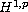Electron. J. Diff. Equ., Vol. 2013 (2013), No. 210, pp. 1-22.

### Homogenization of a system of semilinear diffusion-reaction equations in ansetting Hari Shankar Mahato, Michael Bohm

Abstract:
In this article, homogenization of a system of semilinear multi-species diffusion-reaction equations is shown. The presence of highly nonlinear reaction rate terms on the right-hand side of the equations make the model difficult to analyze. We obtain some a-priori estimates of the solution which give the strong and two-scale convergences of the solution. We homogenize this system of diffusion-reaction equations by passing to the limit using two-scale convergence.

Submitted July 14, 2013. Published September 19, 2013.
Math Subject Classifications: 35B27, 35K57, 35K58, 46E35, 35D30.
Key Words: Global solution; semilinear parabolic equation; reversible reactions; Lyapunov functionals; maximal regularity; homogenization; two-scale convergence.

Show me the PDF file (348 KB), TEX file, and other files for this article.Hari Shankar Mahato Center of Industrial Mathematics, University of Bremen D-28359, Bremen, Germany email: mahato@math.uni-bremen.de Michael Böhm Center of Industrial Mathematics, University of Bremen D-28359, Bremen, Germany email: mbohm@math.uni-bremen.de